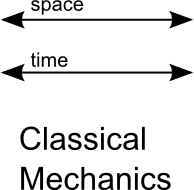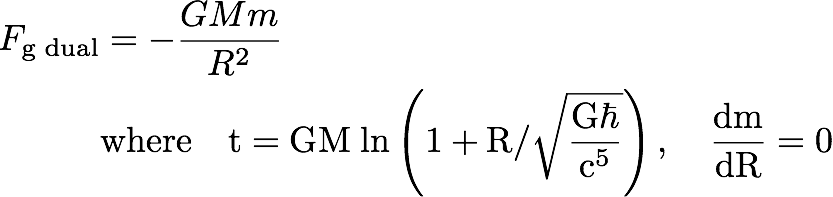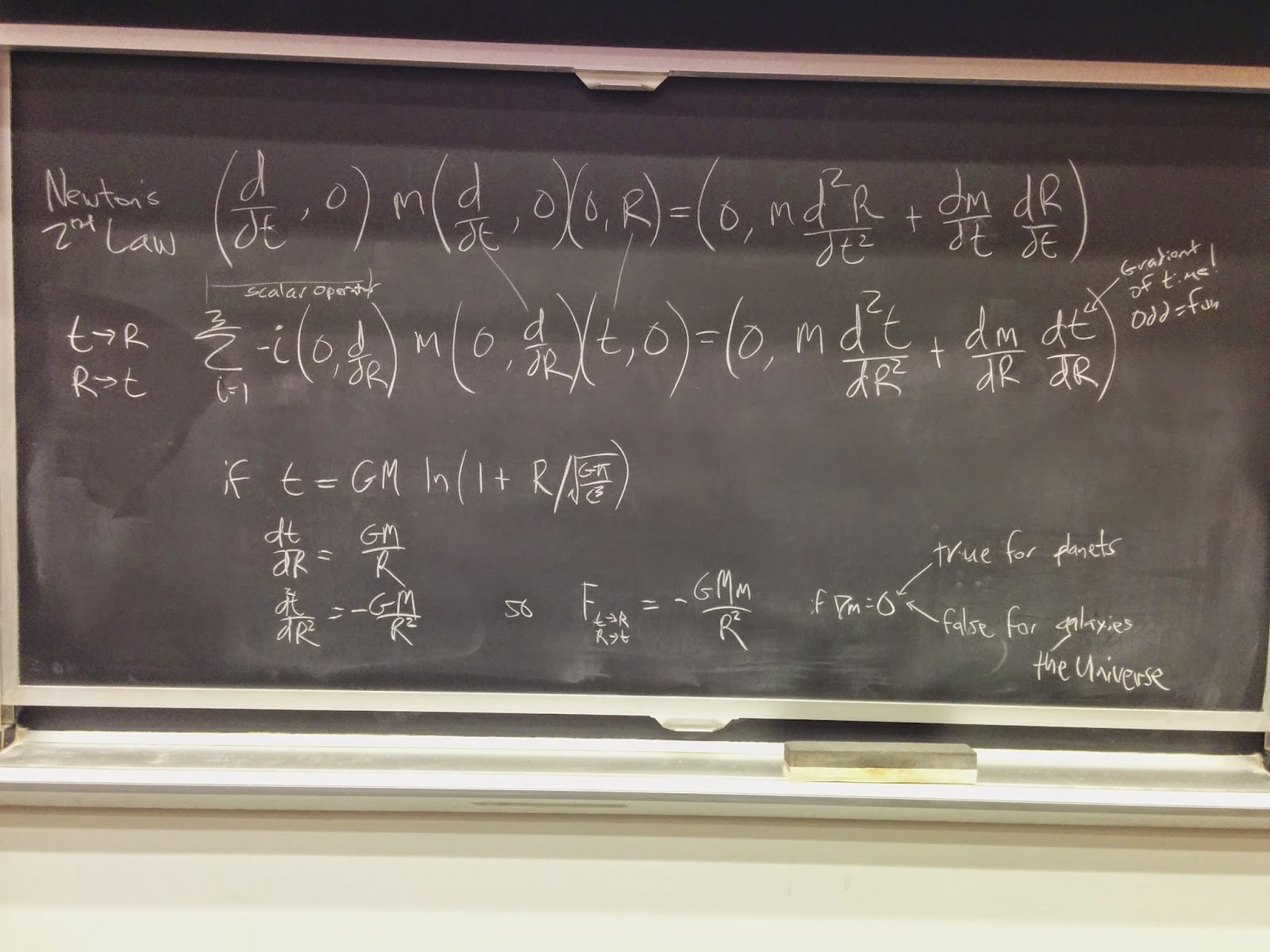I have decided to rescind this proposal.  The swap of time t for space R and visa versa really didn't change anything: a scalar operator was still needed and the single value of time needed three subscripts, not making it look at all like time.  The exact role reversal is too exact.

I do continue to investigate the assumption for self-gravitational system whose mass is distributed over distances of light years that the only effect of gravity is like mA (or mV^2/R for a rotating disk).  The force of gravity may decide not only how fast things go, but where they go.  mA or mV^2/R is about how fast mass goes, but not where mass goes.  Having a "where they go" term on effects side is a non-trivial exercise.  It should be noted that people I respect do not think it is an exercise worth doing.

***

This blog will have a new, testable hypothesis for classical gravity. Start at the beginning of mathematical physics, with work by Newton himself.

I have owned the domain quaternions.com since 1997.  What have I been doing with it all these years?  Not a lot really, the pages have not been updated often. One of the first things I ever did was write Newton's second law on change in inertia as a quaternion expression:[Note: as discussed in the comments below, while this expression happens to have the mA term of Newton's second law, it also has another term that is not invariant under a Galilean transformation. Invariance under a Galilean transformation is a fundamental property of Newton's second law of inertia. For this reason, I have put in a qualifier for references to Newton's second law in the text below.]

What makes this law classical?  When written with quaternions, it is all those zeros.  In this way, classical mechanics treats time separately from space:Newton's second law has been on the books since 1687. Surely I cannot do anything new with the oldest dog in physics.  [Note: the first equation is not Newton's second law, but a significant technical variant.] In December of 2014, I was thinking of this equation again.  In a different reference frame, those zeros would not be zeros.  That would be weird, really weird.  I know what velocity is, dR/dt.  One of the other terms would be The Gradient of Time, dt/dR, which I capitalized because it would make a great name for a jazz band. But it looked like math junk.

A central method in theoretical physics is called duality.  This is where someone starts with something reasonable, say a photon is a wave. That makes all the sense in the world given the study of optics. They then flip it around to claim that a photon is a particle.  Einstein did that with his photon hypothesis in 1905 to explain the photoelectric effect.  A mere 19 years later, De Broglie used duality to justify his hypothesis that particles, the stuff of solids, should also be waves.  That should still sound a bit crazy.  Three years later by bouncing light off of nickel crystals, experimentalist did see wave properties in matter.  People have raked in \$3 million for finding some dualities when working on strings.

Dualities are the domain of modern quantum mechanics, not the Principia.  To teach the oldest dog in physics a new trick, write the [significant variant of the] second law like so:Because of the product rule of calculus, this has the familiar mA term and the less-often used rocket term. [Note: there is only one velocity involved with the product rule, so this is not a rocket equation that needs two velocities: one for the rocket, the other for the fuel.] To construct a dual force hypothesis, use the following two substitutions:

1. t -> Ri

2. Ri -> t

Here is the result:This is easy to write down. It looks unreasonable. That is the nature of a good duality.

I formed a hypothesis.  [The variant of] Newton's second law is the most important law in the classical world we live in.  The dual force would have to be just as important.  If not, it is math junk. I would consider the hypothesis proven wrong.

There are not many candidates in classical physics.  One possibility is Newton's law of gravity:Since the seventeen hundreds, the way physicists write this using a potential is:This is the form physicists use to model gravity for a point source.  Potential theory is always a pairing of the differential equation with the solution to that equation.  The above is a perfect match.  Can a similar pair be found for the dual force hypothesis?The first derivative of t is GM/R. The second derivative ends up with the standard Newtonian gravity force law.

[Note: As pointed out by David Halliday and discussed in more detail in the comment section, this claim is not correct, the proposed solution is wrong because R is made of three separate parts. The substitution of t->R requires thee different t's in the dual force law:We will have to see if people think this is a reasonable solution.]

[Note 2: There was a missing set of parenthesis in the original note.  I think I see what this particular observation is about: it is just a different way of writing a Newtonian gravitational potential as the divergence of a 3-vector.Since this is just a rewrite, it may not be of much interest.]

So long as the small m, the passive gravitational mass, acts as a point, then there is no measurable difference between the standard potential theory and this new approach I will call the dual force for gravity hypothesis.  That is the nature of potential theory: a different pair of differential equations and solutions can have results which cannot be differentiated experimentally. There are an infinite number of equations with potentials that have this property. Nature appears to favor simple expressions. The standard potential theory is simpler because it is first-order. It is the duality with [the variant of] Newton's second law makes the dual force for gravity hypothesis worth exploring.

The dual force equation is a second-order differential equation not a first-order equation.  It is second-order differential equations that appear to rule our Universe: Newton's second law, the Maxwell equations when written with potentials, Schrödinger's equation (for the spatial derivative), and the Klein-Gordon equation.  Moving constantly through space-time is the domain of first-order differential equations.  Change involves second-order differential equations.  Shouldn't the classical equation for gravity be second-order?

What is going on with the units?  Isn't it crazy to do three swaps between space and time and expect to get out a classical law of physics?  As proposed, this "time t" has units of length cubed over time squared.  Take two spatial derivatives (inverse area), multiply by mass, and yes, it has the units of a force, mass times length divided by time squared (mA).  The 1 + R part has to be dimensionless.  Although there is both an hbar and the speed of light c, that does not make this a relativistic quantum gravity hypothesis because those conversion factors are dropped from the final expression.  It is fun that the calling cards of a relativistic quantum gravity theory makes a cameo appearance.

The standard potential theory has had a manageable singularity at the origin.  This has always been unsettling since the potential is a ratio of M/R. There is no issue with the source mass going to zero. Why should distance going to zero behave differently? By using 1+R, the dual force hypothesis travels calmly through zero as R goes to zero just like the source mass. The slopes are both unity at the origin. This is a nice feature of the dual force for gravity hypothesis.

I had a discussion with a small group of students at MIT in January, and here was one of the three blackboards from that talk:I did use quaternions here, having to be a little fancy to get the first operator to be a scalar.

Why would the gradient of the passive gravitational mass not be zero?  Consider a disk galaxy.  The mass falls off exponentially from the center.  The dual force necessarily will make a prediction different from Newtonian potential theory.

My previous blog dealt with three problems with classical gravity. To review one issue, Newtonian potential theory fails to describe the rotation profile of disk galaxies.  There should be a Keplarian decline in the velocity profile, but what is seen is that the velocity stays roughly flat.  Disk galaxies are also unstable mathematically.  The dark matter hypothesis hopes to put in enough stuff to fix both problems.  At this point, we don't know what that stuff is despite our detailed understanding of the standard model.  We don't know where the dark stuff is, although it cannot all be in the plane of the galaxy or the galaxy would be unstable.

Could the dual force hypothesis explain the rotation profile of disk galaxies?  That is an open question.  The dual force hypothesis has no free parameters other than the mass to light ratio (how much stuff is there based on the light seen).  That is a great property for a hypothesis.  At this point, it is a math problem.  Unfortunately, it has proven to be a problem too difficult for me to solve so far. This is astrophysical rocket science, no wonder it is over my head.

I am doing my due diligence.  I own "Galactic Dynamics" by Binney and Tremaine, the "Bible" on the subject.  I can tell you the relevant equation is 2.165.  Mathematica has created the graph on my mac mini that matches figure 2.17 in the good book.  Yet I don't get the math which uses things like modified Bessel functions.  It is frustrating to have a specific hypothesis, but not apply it to the case in question.

The cosmology of the entire Universe should also have a non-zero gradient of the passive gravitational mass.  The passive gravitational mass is everywhere in the Universe.  I have not become familiar with the nuts and bolts of the big bang theory theory to see how this additional term alters the start of the Universe.  I am also clueless into the equations that govern the current expansion of the Universe at an accelerated rate.

The dual force for gravity hypothesis is a new classical equation to govern gravity.  It can repeat every classical calculation done with the standard Newtonian first-order potential theory of gravity. It also should make predictions that are different from that theory if the passive gravitational mass is spread out over light years, as is the case for galaxies, the big bang, and the expansion of the Universe.  The dual force equation must be tested against the data. I like that is it consistent with Newtonian gravity theory for the motions of planets. The dual force fails in the same ways too (light bending, the precession of the perihelion of Mercury, and energy loss by binary pulsars). I have a well-tempered hope for the dual force for gravity hypothesis.

If there are any astrophysical rocket scientists out there, feel free to give the equation a try. Let me know how it goes.

Note to commenters:

This blog will be kept on the dual force for gravity hypothesis.  Should you have a different fix, congratulations.  I will however remove comments that promote the new effort as off-topic for this particular blog.  You are free to write your own blog on this very site.